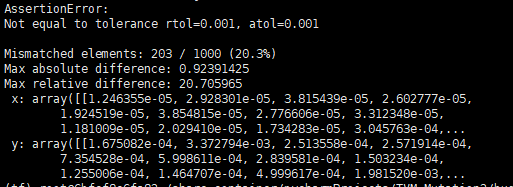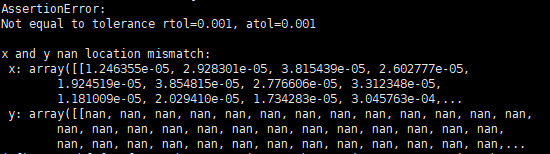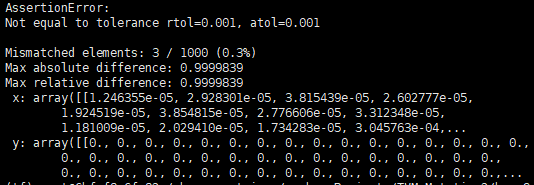# It is opt_level = 4 that causes the compilation to generate a wrong model

Bug description：
I use `opt_level=4` to compile a Keras model. Although the compiled model can be output normally, the predictive ability of the compiled model is lost.

To prove that the compilation error is caused by opt_level in statement `with tvm.transform.PassContext(opt_level=4)`, I changed opt_level to 0,1,2,3, and the compiled model have correct results. Therefore, this bug is related with opt_level =4.

Question : Is this a bug from TVM?

I compared the prediction results of model before and after compilation using the statement np.testing.assert_allclose(res_onnx, res_tvm, rtol=1e-3, atol=1e-3) , and the outputs are as follows. Note that the predict result from compiled model is not fixed, but always wrong！Reproducible script:

``````import keras
import tvm
import tvm.relay as relay
import numpy as np
from PIL import Image
from tvm.contrib import graph_runtime

def image_resize(x, shape):
x_return = []
for x_test in x:
tmp = np.copy(x_test)
img = Image.fromarray(tmp.astype('uint8')).convert('RGB')
img = img.resize(shape, Image.ANTIALIAS)
x_return.append(np.array(img))
return np.array(x_return)

def getTestData():
data_path = "imagenet-val-10.npz"
data = np.load(data_path)
x, y = data['x_test'], data['y_test']
input_shape = [299, 299]
input_precessor = keras.applications.inception_v3.preprocess_input

x_resize = image_resize(np.copy(x), input_shape)
x_test = input_precessor(x_resize)
y_test = keras.utils.to_categorical(y, num_classes=1000)
return x_test, y_test

if __name__ == '__main__':
model_path = 'inception_v3-imagenet_origin.h5'
batch_size = 1
predict_model = keras.models.load_model(model_path)
x_test, y_test = getTestData()
res_frame = predict_model.predict(x_test[:batch_size])

output_shape = (batch_size, 1000)
shape_dict = {'input_6': (batch_size, 3, 299, 299)}
irmod, params = relay.frontend.from_keras(predict_model, shape_dict)

target = 'llvm'
ctx = tvm.cpu(0)

with tvm.transform.PassContext(opt_level=4):
graph, lib, params = relay.build_module.build(irmod, target=target, params=params)
module = graph_runtime.create(graph, lib, ctx)
test_x_tvm = x_test[:batch_size].transpose([0, 3, 1, 2])
data = test_x_tvm.astype('float32')
module.set_input('input_6', data)
module.set_input(**params)
module.run()
res_tvm = module.get_output(0, tvm.nd.empty(output_shape)).asnumpy()

np.testing.assert_allclose(res_frame, res_tvm, atol=1e-3, rtol=1e-3)  # Crash
``````

The Keras model and a small dataset(include 10 pictures) can be downloaded from the below link:

https://drive.google.com/drive/folders/1lFmRq539ptiuYlH4Rw5LeM6r01lsW8l4?usp=sharing

Environment:
TVM : 0.8.dev0
OS: Ubuntu 16.04

Any suggestions would be appreciated. Thanks in advance.

@comaniac @FrozenGene @tqchen @Wheest Can you give me some advice? Thanks.

I ran the given script with only the `AlterOpLayout` and `CombineParallelConv2d` pass enabled and it also outputs incorrect results. But if I enable only either one, it output correct results. That means the error is caused by combination with these passes.

Here is my modification to the script.

``````--- small_bug.py
+++ small_bug.py
@@ -43,7 +43,7 @@ if __name__ == '__main__':
target = 'llvm'
ctx = tvm.cpu(0)

-    with tvm.transform.PassContext(opt_level=4):
+    with tvm.transform.PassContext(opt_level=0, required_pass=["AlterOpLayout", "CombineParallelConv2d"]):
graph, lib, params = relay.build_module.build(irmod, target=target, params=params)
module = graph_runtime.create(graph, lib, ctx)
test_x_tvm = x_test[:batch_size].transpose([0, 3, 1, 2])
``````

Environment: TVM: 0.8.dev0(bf862d) OS: Ubuntu 20.04 on WSL2

1 Like